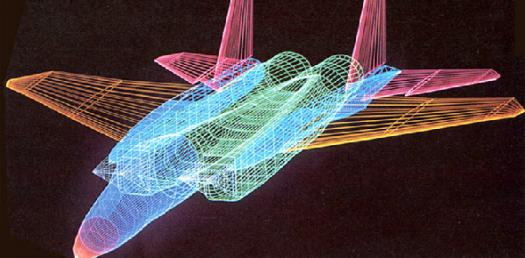# Digital Graphics

18 Questions | Total Attempts: 988SettingsA short multi-choice quiz to test your knowledge regarding the basic characteristics and elements of digital graphics.

• 1.
What is another synonym for graphics?
• A.

Chalk

• B.

Images

• C.

Granite

• 2.
What are the TWO main types of digital graphics?
• A.

Bits (maps) and rasters

• B.

Bitmap (raster) and vector

• C.

Vector and graphites

• 3.
What makes up a raster image?
• A.

Paint

• B.

Pretzels

• C.

Pixels

• 4.
Which term means 'converting an image into a grid of pixels?
• A.

Pixellating

• B.

Pixies

• C.

Rasterizing

• 5.
A vector image is made using a mathematical formula with scalable lines and curves
• A.

True

• B.

False

• 6.
What is image resolution?
• A.

The number of resolutions per inch

• B.

The number of pixels per inch

• C.

The number of lines per inch

• 7.
In relation to image resolution , what does ppi stand for?
• A.

Photographic print image

• B.

Pixels per inch

• C.

Plain photo image

• 8.
In relation to image resolution, what does dpi stand for?
• A.

Dots per inch

• B.

Depth per inch

• C.

Data per inch

• 9.
What image resolution is most common for computer screens?
• A.

350 dpi

• B.

72 dpi

• C.

150 dpi

• 10.
This is an example of an image with low resolution?
• A.

True

• B.

False

• 11.
This is an example of the type of image that would be created using vector?
• A.

True

• B.

False

• 12.
What is the minimum image resolution for printing?
• A.

350 dpi

• B.

72 dpi

• C.

150 dpi

• 13.
Which are the major file formats for raster images?
• A.

PPT

• B.

SVG

• C.

JPEG

• D.

TIFF

• E.

CGM

• F.

BMP

• G.

GIF

• H.

AI

• 14.
Which are examples of file formats for vector images?
• A.

PPT

• B.

SVG

• C.

JPEG

• D.

TIFF

• E.

CGM

• F.

BMP

• G.

GIF

• H.

AI

• 15.
Which file format is best suited for graphics with few colours?
• A.

BMP

• B.

JPEG

• C.

TIFF

• D.

GIF

• 16.
Which software applications would be BEST suited for transforming/creating/manipulating an image?
• A.

Flash CS6

• B.

Excel

• C.

Photoshop

• D.

GIMP

• 17.
Which software applications would be BEST suited for animations, diagrams and simple drawings?
• A.

Flash CS6

• B.

Excel

• C.

Photoshop

• D.

GIMP

• 18.
If you wanted to create a logo to be printed large and small it would be best created as a vector drawing.
• A.

True

• B.

False

Related TopicsBack to top